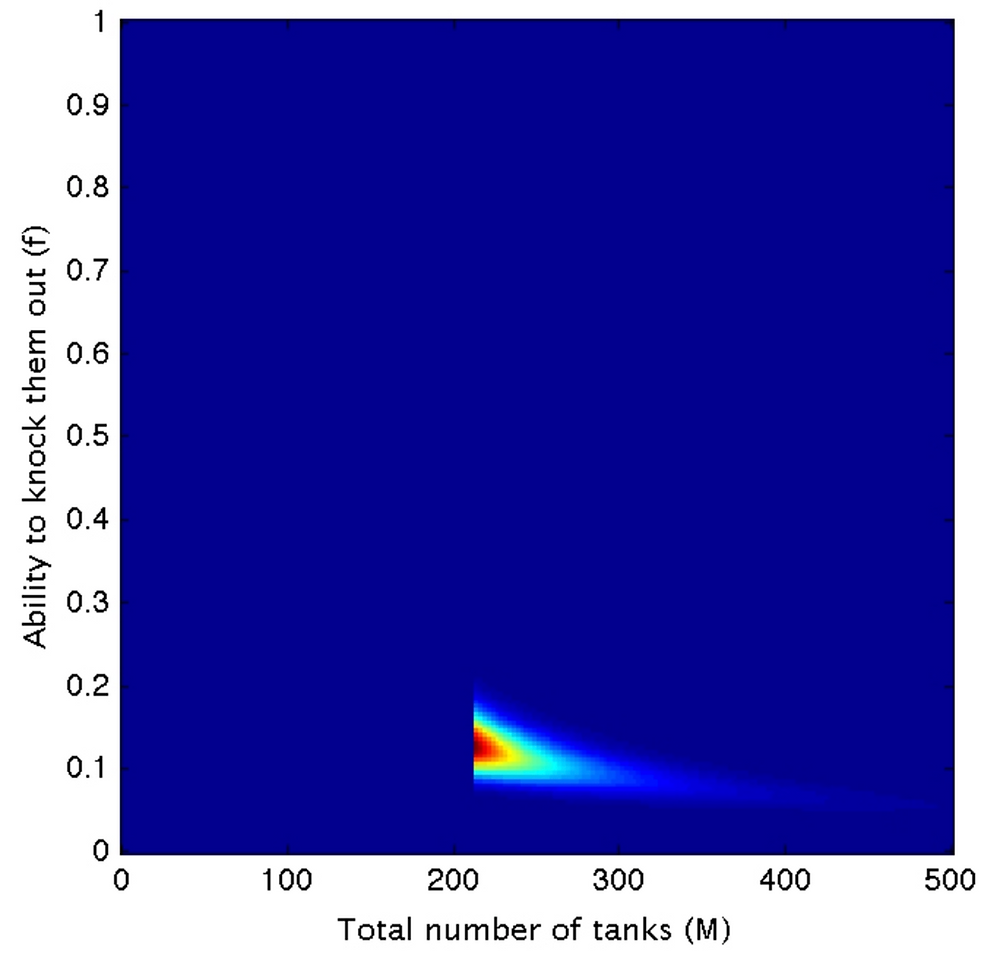Search

# The German Tank Problem (Part II)

Again, a repost of something I wrote about in 2012, the German Tank Problem. This time with a twist. TL:DR - the mathematicians win again.

I've written before about The German Tank Problem. Basically, it's a problem in which you try and estimate the number of tanks being produced by the enemy, based upon the serial numbers of the ones that were knocked out. This was a real problem from World War II, but is now often sold as the taxi problem, in case you are too sensitive to think about tanks.The moral of the story is that the spooks and spies were wrong, and the mathematicians right. Yay!!!

I was happy with this until my post on this (which was almost a year ago). My ex-student, and Bayesian extraordinaire, Brendon Brewer, said something that bothered me. Namely, the chance of seeing a tank depends on the number of tanks, with more chance of seeing one if there are more of them (obviously!), and this seems to cancel out the effect of knowing the serial numbers of the tanks. So how could it work.

Let's start you being a battlefield intelligence officer, and nearby a big battle breaks out and rages. it is too dangerous to venture onto the battle field at the moment, but reports are coming in that there is new, dangerous tank prowling the battlefield. Reports continue, and soldiers report these tanks are everywhere. Reports also come in that the soldiers are knocking out these monsters with an impressive success rate.

Eventually, the battle abates, and you venture on to the battle field, expecting to see heaps of wrecked tanks, but you find far fewer than you expected.

What's happening? If the reports were correct, there were loads of tanks and the soldiers were good and defeating them. So where are all the wrecks?There are two possibilities; either the soldiers were exaggerating the number of tanks that they saw, or their ability to knock them out. Based on what you find, how can you work out the total number of tanks that were present, as this is related to their ability to build new tanks.Let's assume that there were actually M tanks on the battlefield, and the soldiers' abilities meant that they could actually knock a fraction of then, f, out during the battle. The expected number of wrecks on the battlefield is then just λ= f M.

Now we can see the problem! There could have been a small number of tanks, and the soldiers could have been good at knocking them out, but equivalently there could have been a large number of tanks and the soldiers were actually pretty poor at destroying them, or somewhere in between. In all case, the number of wrecks on the battlefield would be the same.

OK, now for the technical part. While λ might be the expected number of wrecks you find, it might not be an integer number. So, how do you relate λ to the probability of seeing a number of wrecks, N, on a battlefield?

The problem was solved long ago, and what you use is the Poisson distribution. If you are not mathematical, the following may look horrible, but if, like me, you don't mind a bit of recreational mathematics, it's rather lovely.Basically, it says that if your expected number is λ, then the chance of you seeing an integer number k is the above expression. A simple example, the number of buses past a bus-stop. The average number in an hour might be 2.6, and so you sit there and could the number in each hourly segment. In the first hour you see two, and then two in the next hour, and then none, and then two, one, three, none, two, two and, more rarely, five buses pass in an hour.

Suppose you saw the wrecks of 27 tanks on the battlefield, what does the probability distribution of f and M look like? Now, this is a 2-dimensional problem, and so I can do it analytically, and, with the help of Matlab, you getThe red is high probability, the blue is low. We have a degeneracy (which is what we knew previously). So, what do you do? Well, last time we discussed this problem, we considered the serial numbers of the knocked out tank. On the battlefield, you take a look at the first tank and its serial number is 123. We know there is at least 123 tanks on the field, and (look back to the previous post if you don't remember), the probability of higher values of the total number of tanks is reduced. So, here's our new probability distribution adding this one serial number.Adding in the next 4 serial numbers (45, 150, 213 & 58) we get thisWe know that there are at least 213 tanks, but the smaller number also helps reduce the probability of larger total numbers of tanks. Going to 15and then the serial numbers of the total number of 27 serial numbers, we get this.Now the probability is concentrated in a little blob, instead of a huge swath of values right across the plane. And what was the input numbers I used for the problem? A total number of tanks being 300, and the ability to knock them out as 0.1. It works :)

Just to do a final check, let's assume that there is only a small number of tanks in total, 34, but that the soldiers are good at knocking them out (f=0.75). What does the probability distribution look like now?Again, everything still works. We see there was a small number of tanks, and that the troops were pretty good at knocking them out (although the uncertainty on this is quite large), but that doesn't matter, as he who has Reverend Bayes on their side has already won the war.

Have a good long weekend!